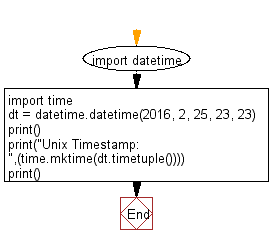﻿ Python: Convert a date to Unix timestamp - w3resource

# Python: Convert a date to Unix timestamp

## Python Datetime: Exercise-35 with Solution

Write a Python program to convert a date to a Unix timestamp.

Sample Solution:

Python Code:

``````import datetime
import time
dt = datetime.datetime(2016, 2, 25, 23, 23)
print()
print("Unix Timestamp: ",(time.mktime(dt.timetuple())))
print()
```
```

Sample Output:

```Unix Timestamp:  1456422780.0
```

Flowchart:Python Code Editor: